## Overview

In this paper, we propose a new generic fairness learning paradigm, called fairness reprogramming: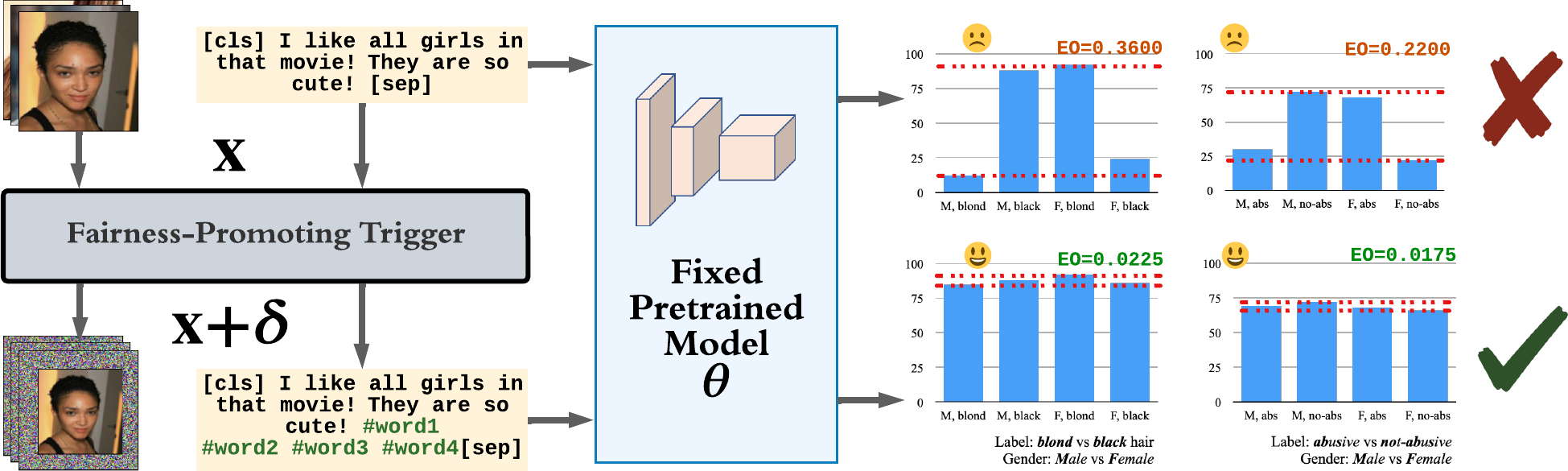Figure 1. An example of fairness reprogramming in CV and NLP tasks. The input agnostic trigger can promote fairness without altering the pretrained model.

## Principal Research Question

Can an unfair model be reprogrammed into a fair one?
If so, why and how would it work?

## Fairness Reprogramming

Consider a classification task, where $$\mathbf{X}$$ represents the input feature and $$Y$$ represents the output label. There exists some sensitive attributes or demographic groups, $$Z$$, that may be spuriously correlated with $$Y$$. There is a pre-trained classifier, $$f^*(\cdot)$$ that predicts $$Y$$ from $$\mathbf{X}$$, i.e., $$\hat{Y} = f^*(\mathbf{X})$$.

The goal of fairness reprogramming is to improve the fairness of the classifier by modifying the input $$\mathbf{X}$$, while keeping the classifier’s weights $$\boldsymbol\theta$$ fixed. In particular, we aim to achieve either of the following fairness criteria.

• Equalized Odds:
$\hat{Y} \perp Z | Y,$
• Demographic Parity:
$\hat{Y} \perp Z,$

where $$\perp$$ denotes independence.

### Fairness Trigger

The reprogramming primarily involves appending a fairness trigger to the input. Formally, the input modification takes the following generic form:

$\tilde{\mathbf{X}} = m(\mathbf{X}; \boldsymbol\theta, \boldsymbol \delta) = [\boldsymbol \delta, g(\mathbf{X}; \boldsymbol\theta)],$

where $$\tilde{\mathbf{X}}$$ denotes the modified input; $$[\cdot]$$ denotes vector concatenation (see Figure 1).

### Optimization Objective and Discriminator

Our optimization objective is as follows

$\min_{\boldsymbol\theta, \boldsymbol\delta} \,\,\, \mathcal{L}_{\text{util}} (\mathcal{D}_{\text{tune}}, f^* \circ m) + \lambda \mathcal{L}_{\text{fair}} (\mathcal{D}_{\text{tune}}, f^* \circ m),$

where $$\mathcal{D}_{\text{tune}}$$ represents the dataset that is used to train the fairness trigger. The first loss term, $$\mathcal{L}_{\text{util}}$$, is the utility loss function of the task. For classification tasks, $$\mathcal{L}_{\text{util}}$$ is usually the cross-entropy loss, i.e.,:

$\mathcal{L}_{\text{util}}(\mathcal{D}_{\text{tune}}, f^* \circ m) = \mathbb{E}_{\mathbf{X}, Y \sim \mathcal{D}_{\text{tune}}} [\textrm{CE}(Y, f^*(m(\mathbf{X})))],$

The second loss term, $$\mathcal{L}_{fair}$$, encourages the prediction to follow the fairness criteria and should measure how much information about $$Z$$ is in $$\hat{Y}$$. Thus, we introduce another network, the discriminator, $$d(\cdot; \boldsymbol \phi)$$, where $$\boldsymbol \phi$$ represents its parameters. If the equalized odds criterion is applied, then $$d(\cdot; \boldsymbol \phi)$$ should predict $$Z$$ from $$\hat{Y}$$ and $$Y$$; if the demographic parity criterion is applied, then the input to $$d(\cdot; \boldsymbol \phi)$$ would just be $$\hat{Y}$$. The information of $$Z$$ can be measured by maximizing the negative cross-entropy loss for the prediction of $$Z$$ over the discriminator parameters:

$\mathcal{L}_{\text{fair}} (\mathcal{D}_{\text{tune}}, f^* \circ m) = \max_{\boldsymbol \phi} \mathbb{E}_{\mathbf{X}, Y, Z \sim \mathcal{D}_{\text{tune}}} [-\textrm{CE}(Z, d(f^*(m(\mathbf{X})), Y; \boldsymbol \phi))].$

We give an illustration of our fairness reprogramming algorithm below, which co-optimizes the fairness trigger and the discriminator at the same time in a min-max fashion.Figure 2. An illustration of our proposed fairness reprogramming algorithm.

## Experiment results

We consider the following two commonly used NLP and CV datasets:

• Civil Comments: The dataset contains 448k texts with labels that depict the toxicity of each input. The demographic information of each text is provided.

• CelebA: The dataset contains over 200k human face images and each contains 39 binary attribute annotations. We adopt the hair color prediction task in our experiment and use gender annotation as the demographic information.Figure 3. Results on (a) Civil Comments and (b) CelebA. We report the negative DP (left) and the negative EO (right) scores. For each method, we vary the trade-off parameter λ to record the performance. The closer a dot is to the upper-right corner, the better the model is.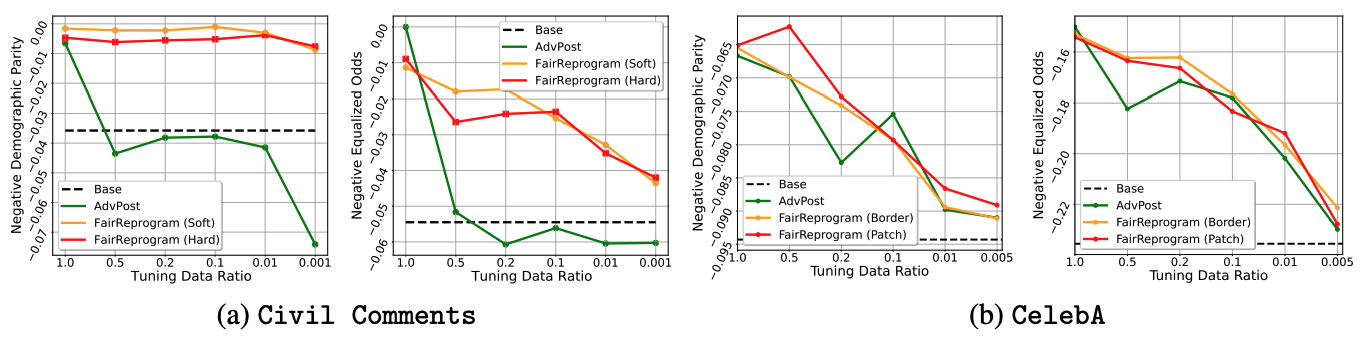Figure 4. Results on (a) Civil Comments and (b) CelebA with different tuning data ratios. We report the negative DP (left) and negative EO (right) scores. We consider a fixed BASE model trained with a training set, whose negative bias scores are presented as a black dashed line. Then we train other methods with different tuning data ratios to promote fairness of the BASE model.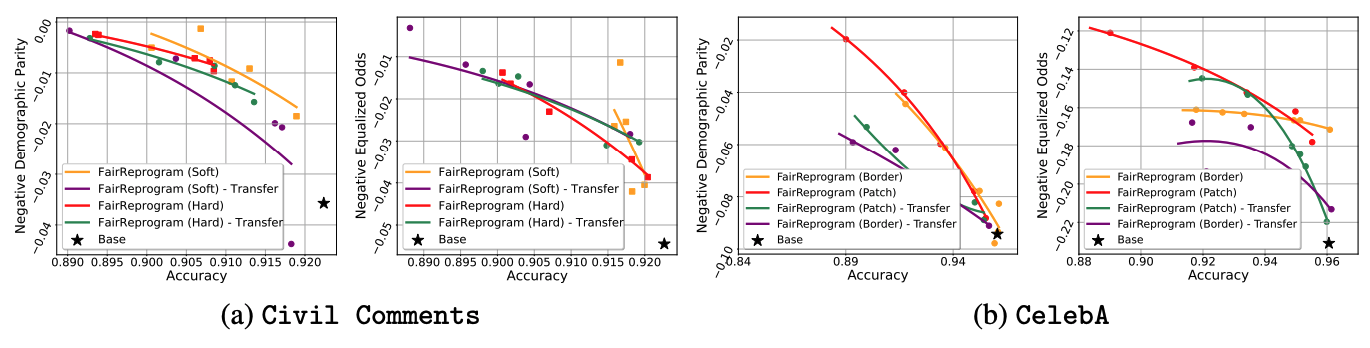Figure 5. Results in the transfer setting. We report negative DP (left) and negative EO (right) scores. The triggers are first trained in a BASE model. Then, we evaluate the triggers based on another unseen BASE model. We change the parameter λ to trade off accuracy with fairness and draw the curves in the same way as Figure 3.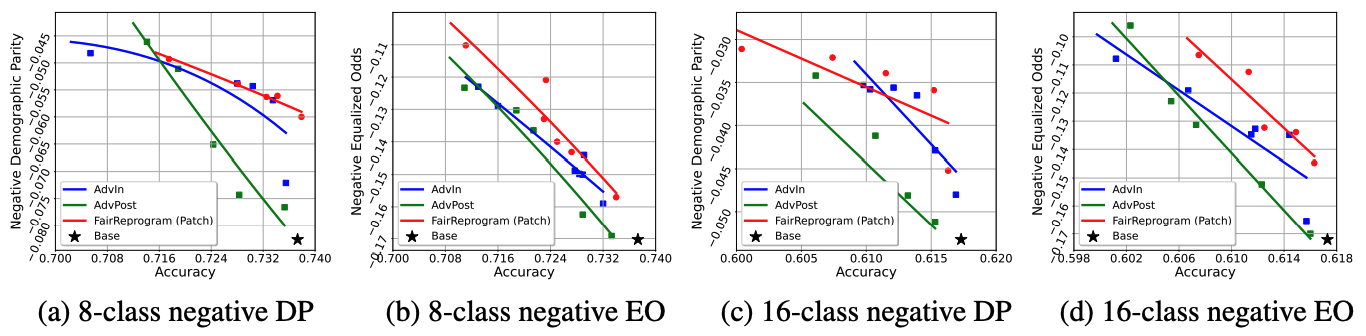Figure 6. Performance of multi-class classification. For (a) and (b), we use the attributes Blond Hair, Smiling, and Attractive for multi-class construction. We add an additional attribute Wavy Hair for (c) and (d).

## Why does Fairness Trigger work?

In our paper, we both theoretically prove and empirically demonstrate why a global trigger can obscure the demographic information for any input. In general, the trigger learned by the reprogrammer contains very strong demographic information and blocks the model from relying on the real demographic information from the input. Since the same trigger is attached to all the input, the uniform demographic information contained in the trigger will weaken the dependence of the model on the true demographic information contained in the data, and thus improve the fairness of the pretrained model.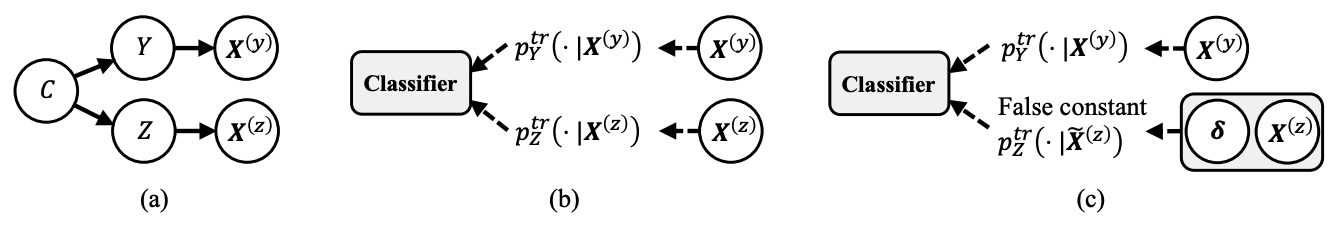Figure 7. Illustration of why fairness trigger works. (a) The data generation process. (b) The information flow from data to the classifier through sufficient statistics. (c) A fairness trigger strongly indicative of a demographic group can confuse the classifier with a false demographic posterior, thus preventing the classifier from using the correct demographic information.

### Input Saliency Analysis

The following two figures compare the saliency maps of some example inputs with and without the fairness triggers. Specifically, For the NLP applications, we extract a subset of Civil Comments with religion-related demographic annotations, and apply IG to localize word pieces that contribute most to the text toxicity classification. For the CV application, we use GradCam to identify class-discriminative regions of CelebA’s test images.

Figure 8 presents the input saliency maps using GradCam1 on two input images with respect to their predicted labels, non-blond hair and blond hair, respectively. When there is no fairness trigger, the saliency region incorrectly concentrates on the facial parts, indicating the classifier is likely to use biased information, such as gender, for its decision. With the fairness trigger, the saliency region moves to the hair parts.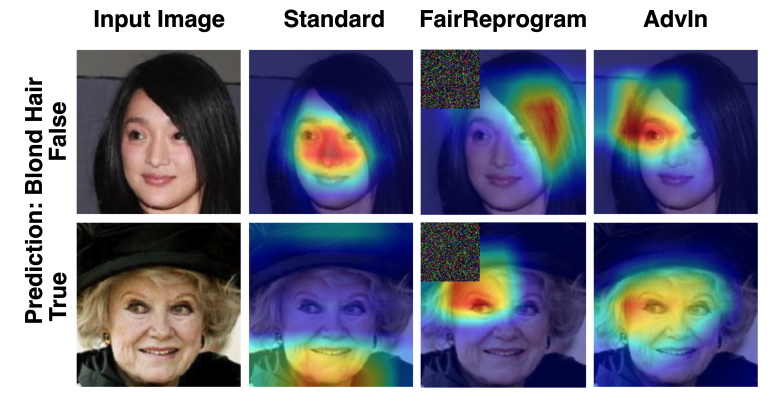Figure 8. Gradient-based saliency map visualized with GradCam of different methods. The highlighted zones (marked in red) depicting regions exerting major influence on the predicted labels (non-blond hair v.s. blond hair) in each row, which also depict the attention of the model on the input image.

In Figure 9, through Integrated Gradient23 we show that our fairness trigger consists of a lot of religion-related words (e.g., diocesan, hebrew, parish). Meanwhile, the predicted toxicity score of the benign text starting from ‘muslims’ significantly reduces. These observations verify our theoretical hypothesis that the fairness trigger is strongly indicative of a certain demographic group to prevent the classifier from using the true demographic information.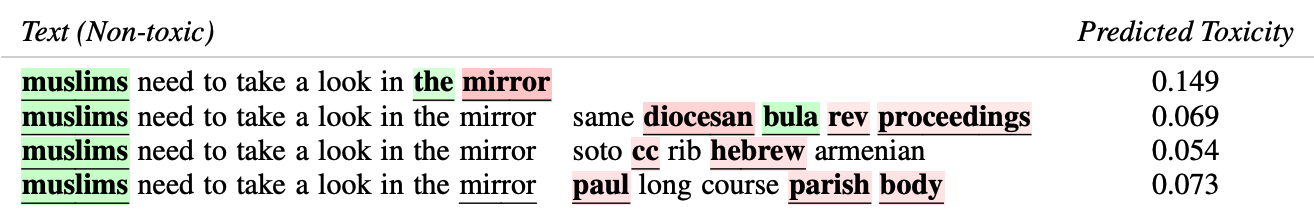Figure 9. A text example from Civil Comments with Integrated Gradient highlighting important words that influence ERM model predictions. The text is concatenated with three triggers generated with different adversary weights. Green highlights the words that lean toward toxic predictions and red highlights non-toxic leaning words. The model prediction tends to be correct after adding the triggers.

To further verify that the triggers encode demographic information, we trained a demographic classifier to predict the demographics from the input (texts or images). We use the demographic classifier to predict the demographic information of a null image/text with the trigger. We see that the demographic classifier gives confident outputs on the triggers, indicating that they found triggers are highly indicative of demographics.Figure 10. Predictions of the demographic classifier on a null input with triggers generated by different λ. The demographic prediction for CV triggers indicate the predicted score for Male and Female, and it is Christian, Muslim, and other religion for NLP.

## Citation

@inproceedings{zhang2022fairness,
title = {Fairness reprogramming},
author = {Zhang, Guanhua and Zhang, Yihua and Zhang, Yang and Fan, Wenqi and Li, Qing and Liu, Sijia and Chang, Shiyu},
booktitle = {Advances in Neural Information Processing Systems},
year = {2022}
}


## Reference

 Ramprasaath R Selvaraju et al. “Grad-cam: Visual explanations from deep networks via gradient-based localization” ICCV 2017.

 Mukund Sundararajan et al. “Axiomatic attribution for deep networks” ArXiv, vol. abs/1703.01365, 2017.

 Narine Kokhlikyan et al. “Captum: A unified and generic model interpretability library for PyTorch” arXiv preprint arXiv:2009.07896.